• estimator =KMeans(n_clusters=3,init = 'kmeans++') #构造一个聚类数为5的聚类器,初始质心选取方式改为kmeans++ estimator.fit(data1) #聚类 label_pred = estimator.labels_ #获取聚类标签 centroids = estimator....
estimator =KMeans(n_clusters=3,init = 'kmeans++')   #构造一个聚类数为5的聚类器,初始质心选取方式改为kmeans++
estimator.fit(data1)   #聚类
label_pred = estimator.labels_  #获取聚类标签
centroids = estimator.cluster_centers_ #获取聚类中心
print(label_pred)
#画图，每个类别画出一条线
for  i in range(len(label_pred)):
if label_pred[i] == 0:
x = [i for i in range(len(data1))]
plt.plot(x, data1[i], 'y')
elif label_pred[i] == 1:
x = [i for i in range(len(data1))]
plt.plot(x, data1, 'b')
else:
x = [i for i in range(len(data1))]
plt.plot(x, data1[i], 'r')

显示列索引
x = df.columns.values.tolist()


展开全文• 前言 KMedoids的聚类有时比KMeans的聚类效果要好。... Python (>=3.6) scikit-learn(>=0.22) 安装 scikit-learn-extra PyPi: pip install scikit-learn-extra Conda: conda install -c
前言

KMedoids的聚类有时比KMeans的聚类效果要好。手上正好有一批时序数据，今天用KMedoids试下聚类效果

安装

KMedoids可以使用sklearn的拓展聚类模块scikit-learn-extra，模块需要保证

Python (>=3.6)

scikit-learn(>=0.22)

安装 scikit-learn-extra

PyPi: pip install scikit-learn-extra
Conda: conda install -c conda-forge scikit-learn-extra
Git: pip install https://github.com/scikit-learn-contrib/scikit-learn-extra/archive/master.zip

安装 tslearn

PyPi: python -m pip install tslearn
Conda: conda install -c conda-forge tslearn
Git: python -m pip install https://github.com/tslearn-team/tslearn/archive/master.zip

为什么要使用scikit-learn-extra和tslearn两个模块？因为sklearn里面没有自带的KMedoids，也没有类似DTW的时序度量算法，但组合两者恰好能解决问题

测试

import numpy as np
from sklearn_extra.cluster import KMedoids
import tslearn.metrics as metrics
# 自定义数据处理
import data_process
from tslearn.clustering import silhouette_score
from tslearn.preprocessing import TimeSeriesScalerMeanVariance
from tslearn.generators import random_walks
from sklearn.preprocessing import StandardScaler
import matplotlib.pyplot as plt

# shape(X) = (100,2800+)
X = np.loadtxt("top100.txt",dtype=np.float,delimiter=",")
# 降成30维
X = data_process.downsample(X,30)
seed = 0

# elbow法则找最佳聚类数，结果：elbow = 5
def test_elbow():
global X,seed
distortions = []
dists = metrics.cdist_dtw(X) # dba + dtw
# dists = metrics.cdist_soft_dtw_normalized(X,gamma=.5) # softdtw
for i in  range ( 2 , 15 ):
km = KMedoids(n_clusters=i,random_state=seed,metric="precomputed")
km.fit(dists)
#记录误差和
distortions.append(km.inertia_)
plt.plot(range ( 2 , 15 ), distortions, marker= 'o' )
plt.xlabel( 'Number of clusters' )
plt.ylabel( 'Distortion' )
plt.show()

def test_kmedoids():

num_cluster = 5
# 声明precomputed自定义相似度计算方法
km = KMedoids(n_clusters= num_cluster, random_state=0,metric="precomputed")
# 采用tslearn中的DTW系列及变种算法计算相似度，生成距离矩阵dists
dists = metrics.cdist_dtw(X) # dba + dtw
# dists = metrics.cdist_soft_dtw_normalized(X,gamma=0.5) # softdtw
y_pred = km.fit_predict(dists)
np.fill_diagonal(dists,0)
score = silhouette_score(dists,y_pred,metric="precomputed")
print(X.shape)
print(y_pred.shape)
print("silhouette_score: " + str(score))
for yi in range(num_cluster):
plt.subplot(3, 2, yi + 1)
for xx in X[y_pred == yi]:
plt.plot(xx.ravel(), "k-", alpha=.3)
# 注意这里的_cluster_centers要写成X[km.medoid_indices_[yi]]，因为你是precomputed，源码里面当precomputed时_cluster_centers等于None
plt.plot(X[km.medoid_indices_[yi]], "r-")
plt.text(0.55, 0.85,'Cluster %d' % (yi + 1),
transform=plt.gca().transAxes)
if yi == 1:
plt.title("KMedoids" + " + DBA-DTW")

plt.tight_layout()
plt.show()

#test_elbow()
test_kmedoids()

采用KMedoids + DBA-DTW聚类效果  # 轮廓系数silhouette_score: 0.5465097470777784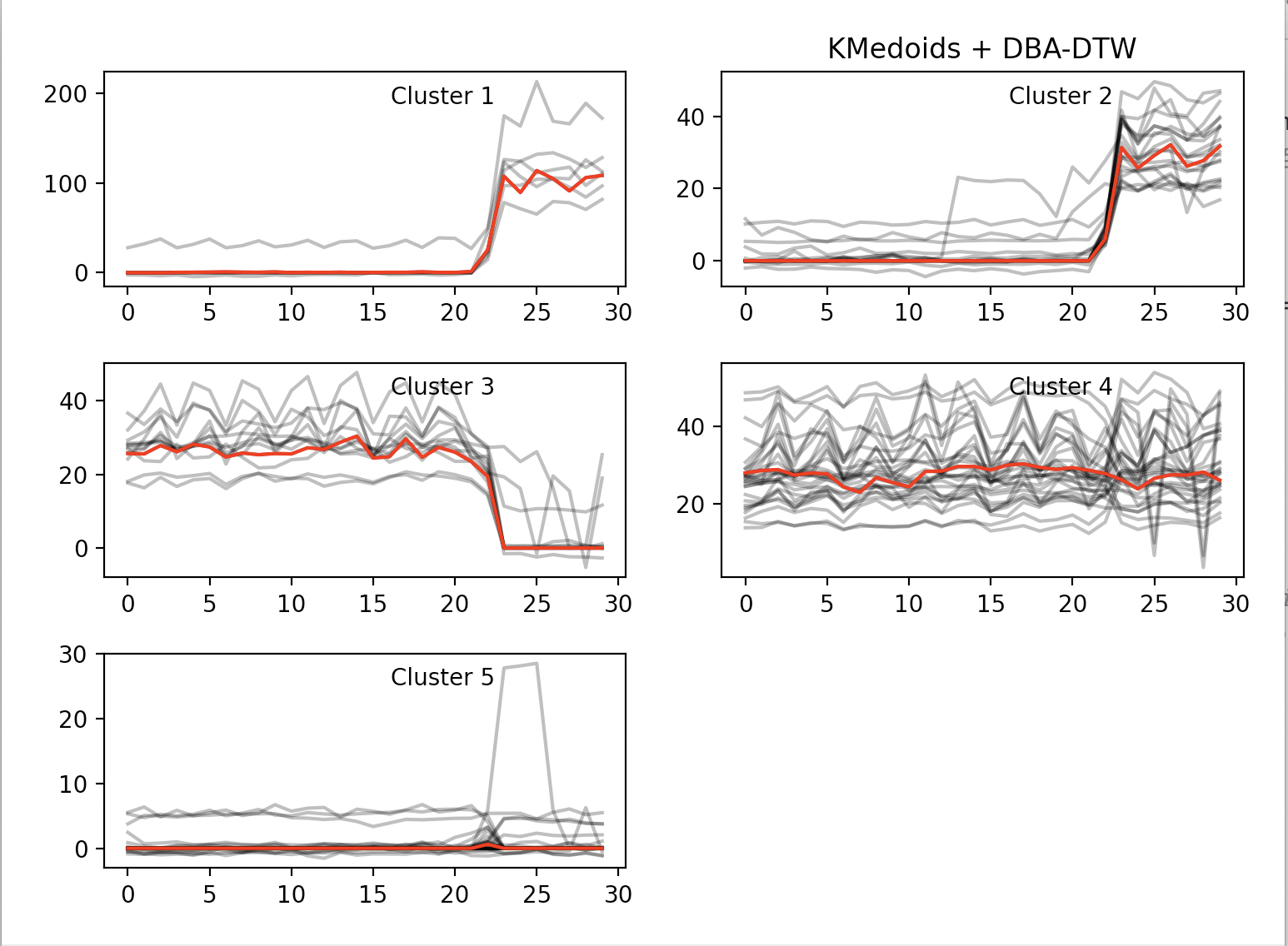采用KMedoids + SoftDTW聚类效果   # 轮廓系数silhouette_score: 0.6528261125440392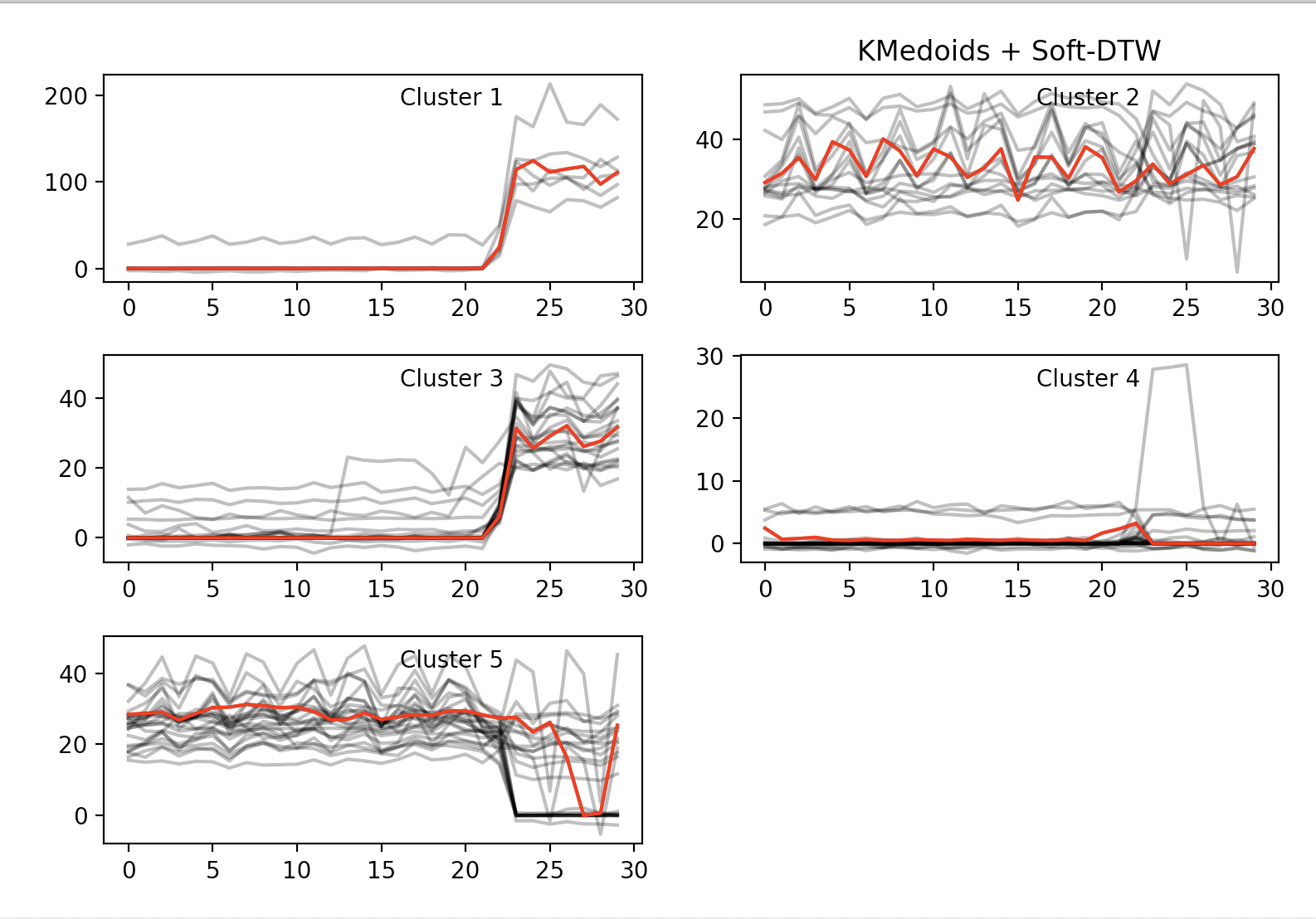直接采用欧氏距离的聚类效果    # 轮廓系数silhouette_score: 0.5209641775604567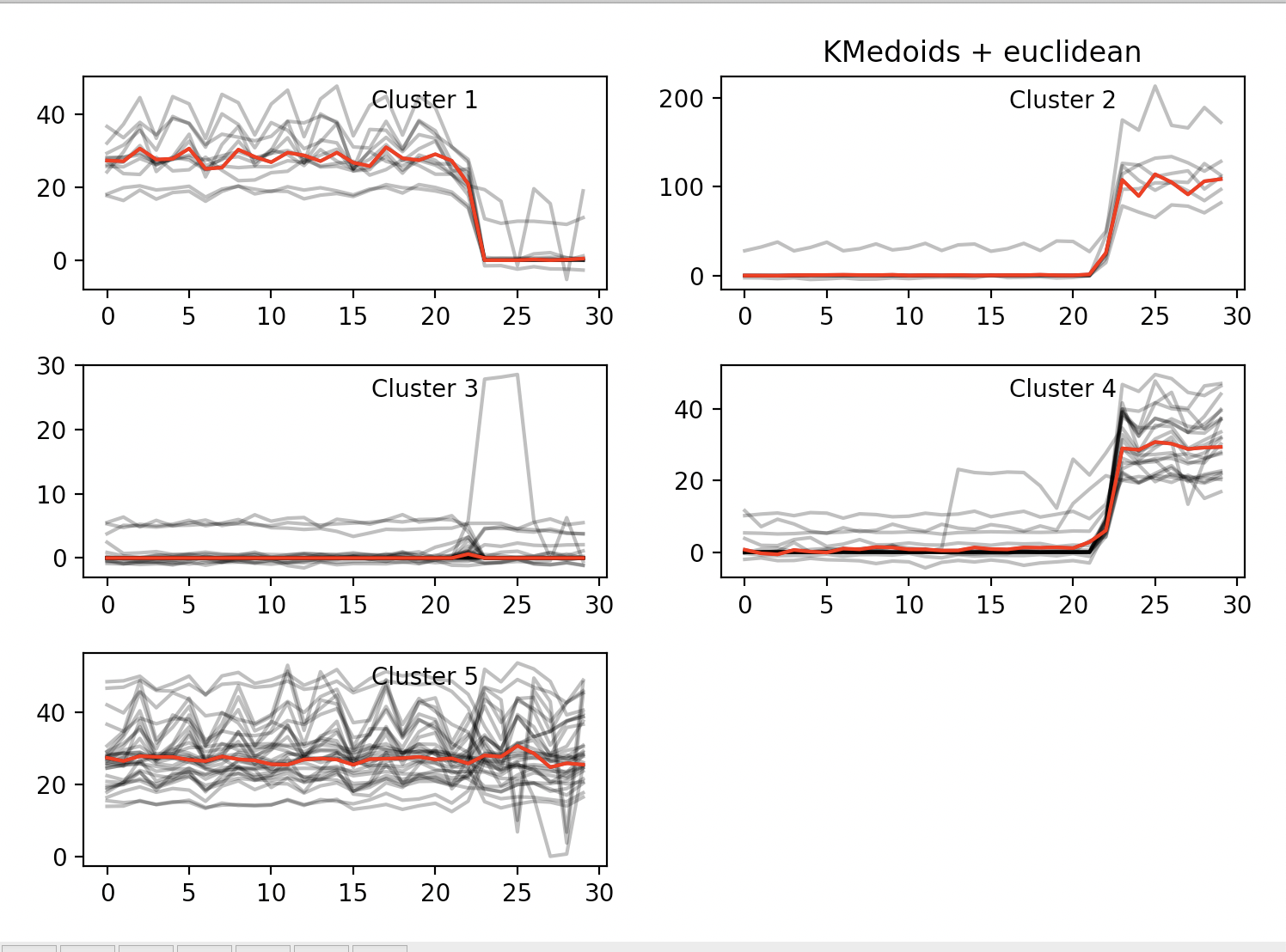相比采用KMeans，KMedoids在的聚类中心（红线部分）从视觉上要似乎要更好（KMeans+DTW聚类效果可见该文章最后一节），但轮廓系数却不如KMeans。但仅欧氏距离而言，KMedoids的轮廓系数要比KMeans更好那么一点

展开全文• 2019年神经信息处理系统（NeurIPS）接受了论文“时间序列聚类的学习表示形式”。 郑家伟和李森同样为这项工作做出了贡献。 代码 DTCR的代码。 依存关系 tensorflow 1.0及更高版本 用法 该存储库包含UCR数据集中的...
• 用于时间序列聚类的端到端深度表示学习 这是与为“时间序列聚类的端到端深度表示学习：一个比较研究”（Baptiste Lafabregue，Jonathan Weber，PierreGançarki和Germain Forestier）所做的实验相对应的代码 数据集 ...
• 离散控制Matlab代码Syntgen：具有聚类和已知地面事实的合成时态网络生成器 描述 请参阅IMA复杂网络杂志上发布的“ Syntgen：一种使用用户指定的拓扑生成时态网络的系统”，以进行网络生成。 请参阅IEEE Xplore存储库...
• 时间序列数据聚类方法python代码（k-means) import numpy import matplotlib.pyplot as plt from tslearn.clustering import TimeSeriesKMeans from tslearn.datasets import CachedDatasets from tslearn....
          时间序列数据聚类方法python代码（k-means)

import numpy
import matplotlib.pyplot as plt
from tslearn.clustering import TimeSeriesKMeans
from tslearn.datasets import CachedDatasets
from tslearn.preprocessing import TimeSeriesScalerMeanVariance, TimeSeriesResampler
seed = 0
numpy.random.seed(seed)
X_train, y_train, X_test, y_test = CachedDatasets().load_dataset(“Trace”)
X_train = X_train[y_train < 4]  # Keep first 3 classes
numpy.random.shuffle(X_train)
X_train = TimeSeriesScalerMeanVariance().fit_transform(X_train[:50])  # Keep only 50 time series
X_train = TimeSeriesResampler(sz=40).fit_transform(X_train)  # Make time series shorter
sz = X_train.shape
Euclidean k-means
print(“Euclidean k-means”)
km = TimeSeriesKMeans(n_clusters=3, verbose=True, random_state=seed)
y_pred = km.fit_predict(X_train)
plt.figure()
for yi in range(3):
plt.subplot(3, 3, yi + 1)
for xx in X_train[y_pred == yi]:
plt.plot(xx.ravel(), “k-”, alpha=.2)
plt.plot(km.cluster_centers_[yi].ravel(), “r-”)
plt.xlim(0, sz)
plt.ylim(-4, 4)
if yi == 1:
plt.title(“Euclidean $k$-means”)
DBA-k-means
print(“DBA k-means”)
dba_km = TimeSeriesKMeans(n_clusters=3, n_init=2, metric=“dtw”, verbose=True, max_iter_barycenter=10, random_state=seed)
y_pred = dba_km.fit_predict(X_train)
for yi in range(3):
plt.subplot(3, 3, 4 + yi)
for xx in X_train[y_pred == yi]:
plt.plot(xx.ravel(), “k-”, alpha=.2)
plt.plot(dba_km.cluster_centers_[yi].ravel(), “r-”)
plt.xlim(0, sz)
plt.ylim(-4, 4)
if yi == 1:
plt.title(“DBA $k$-means”)
Soft-DTW-k-means
print(“Soft-DTW k-means”)
sdtw_km = TimeSeriesKMeans(n_clusters=3, metric=“softdtw”, metric_params={“gamma_sdtw”: .01},
verbose=True, random_state=seed)
y_pred = sdtw_km.fit_predict(X_train)
for yi in range(3):
plt.subplot(3, 3, 7 + yi)
for xx in X_train[y_pred == yi]:
plt.plot(xx.ravel(), “k-”, alpha=.2)
plt.plot(sdtw_km.cluster_centers_[yi].ravel(), “r-”)
plt.xlim(0, sz)
plt.ylim(-4, 4)
if yi == 1:
plt.title(“Soft-DTW $k$-means”)
plt.tight_layout()
plt.show()


展开全文• 可靠的方法去判断两个时间序列是否相似，截下来便可以使用k-NN算法进行分类。根据经验，最优解一般出现在k=1的时候。下面就利用DTW欧氏距离的1-NN算法。在该算法中，train是时间序列示例的训练集，其中时间序列所属...
• 2017年KDD最佳论文，解决高维时间信号的自动分割聚类问题，并给出基于python的源码。

Hallac, David, et al. “Toeplitz Inverse Covariance-Based Clustering of Multivariate Time Series Data.” KDD. (2017).

本文是2017年KDD最佳论文，解决了高维时间信号的自动分割聚类问题，并给出了基于python的源码（戳这里下载）。
核心思想
在实际问题中有大量随时间变化的高维信号。
驾驶汽车：油门，刹车，地理位置，空调，门窗…
支付软件：用户的登陆，转入，提现，消费…
在没有标定的情况下，想发掘这些数据中隐藏的信息，即将信号划分为若干可能的状态，并标记每条信号的各段。
驾驶汽车：起步，上坡，超车，拥堵…
支付软件：用户发薪，过节，打新股…
这就需要同时对数据进行两种操作：
将各条数据进行分割
将分割结果各个聚类
传统聚类方法考察信号各维度的绝对值，以确定信号间的相似度；
本文算法考察信号各维度之间的相关性，以确定信号间的相似度。
建模
信号段
设有时间长度为TT的原始信号x=[x1,x2...xT]\textbf {x}=\left[ x_1, x_2...x_T\right]

实际应用中，会有来自多个用户的多段原始信号。这里可以直接将它们连缀成一个向量。如果需要考虑绝对时刻，则可以将时间戳也作为一维信号增补上去。

其中每一时刻的信号xix_i为nn维向量。
为了便于考察信号相关性，以每一时刻为基准，向前截取宽度为ww的一段：Xi=[xi−w+1,...xi−1,xi]X_i=\left[ x_{i-w+1},...x_{i-1}, x_i\right]
i=1,2...Ti=1,2...T
信号段XiX_i为nwnw维向量。
类别信息
预期将所有信号段划分为KK类，属于第jj类的信号段序号集合记为Pj,j=1,2...KP_j, j=1,2...K。

举例：有信号段ABAAC，n=5n=5, K=3K=3。P1=[0,2,3],P2=,P3=P_1=[0, 2, 3], P_2=, P_3=

认为每一类信号段服从0均值高斯分布，其协方差逆矩阵为Θj,j=1,2...K\Theta_j, j=1,2...K。这是一个nw×nwnw \times nw的矩阵。
Θi\Theta_i由w×ww\times w个子矩阵组成，每个子矩阵的尺寸为n×nn\times n。位置pqpq的子矩阵描述时刻pp和时刻qq之间，nn个维度之间的协方差逆矩阵。[0−w+1,...p,...q,...0][0-w+1, ... p, ...q, ...0]
这里假设信号是非时变的，不同时刻信号之间的关系只和相对时间差有关；交换pq，其协方差逆阵互为转置。
换句话说，Θj\Theta_j是个分块Toeplitz矩阵：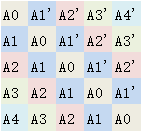每条斜线上的子矩阵相同；对角对称斜线的子矩阵互为转置。
求解
我们需要轮流求解两个问题
给定Θj\Theta_j，求解信号段分类方法PjP_j
给定PjP_j，求解各类逆协方差阵Θj\Theta_j
信号段分类PjP_j
给定Θj\Theta_j，把信号段XiX_i归入jj类的代价可以负对数似然表示：E(i∈Pj)=−ll(i,j)=−log⁡[N(Xi;0,Θj−1)]E(i\in P_j)=-ll(i,j)=-\log \left[ N(X_i;0,\Theta_j^{-1})\right]
=−log⁡[det⁡(Θj)1/2⋅exp⁡[−12XiTΘjXi]]=−log⁡det⁡(Θj)+XiTΘjXi=-\log \left[ \det(\Theta_j)^{1/2} \cdot \exp \left[ -\frac{1}{2}X_i^T\Theta_jX_i\right] \right]=-\log \det(\Theta_j) + X_i^T\Theta_jX_i
另外考虑信号的连续性：相连信号段不同类时施加惩罚β\beta。E(i,i+1)={0i,i+1同类βi,i+1不同类E(i,i+1)=\begin{cases}0 & i,i+1同类\\ \beta & i,i+1不同类\end{cases}
两个代价构成经典的流水线调度问题，可以使用BP算法求解。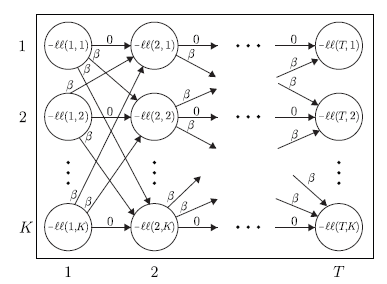其核心思路是：在给第i个信号段分类时，只需考虑第i-1信号段分为各类时的代价即可。
逆协方差阵Θj\Theta_j
给定一类中所有信号段集合PjP_j，通过最小化其负对数似然总和，可以求解Θj\Theta_j。
各类可以并行计算，故书写时省去下标j。
E(Θ)=∑i∈P−ll(Xi,Θ)=∑i∈P−log⁡det⁡(Θ)+XiTΘXi=E1+E2E(\Theta)=\sum_{i \in P} -ll(X_i, \Theta)=\sum_{i \in P} -\log \det(\Theta) + X_i^T\Theta X_i=E_1 + E_2
求和号内第一项和jj无关，∣∣||表示集合内元素计数：E1=−∣Pi∣⋅log⁡det⁡(Θ)E_1=-|P_i|\cdot \log \det (\Theta)
求和号内第二项可以写成迹的形式：E2=−tr(∑i∈PXiTXi)=−∣Pi∣⋅tr(S⋅Θ)E_2=-tr(\sum_{i\in P}X_i^TX_i)=-|P_i|\cdot tr(S\cdot \Theta)
其中SS是由PjP_j中所有信号段计算得到的当前协方差阵。1
另外添加一个正则项：E3=∣∣λ⊙Θ∣∣1E_3=||\lambda \odot \Theta||_1
其中λ\lambda为权重矩阵，⊙\odot表示矩阵对位相乘。
根据前述，要求Θ\Theta是分块Toeplitz矩阵。
把问题稍作变换为如下形式，可以用ADMM2算法快速求解：minimize −log⁡det⁡Θ+tr(S⋅Θ)+∥∣λ⊙Z∣∣1minimize\ -\log \det \Theta+tr(S\cdot \Theta) + \||\lambda\odot Z||_1
subject to Θ=Z,Z为分块Toeplitz矩阵subject\ to\ \Theta=Z, Z为分块Toeplitz矩阵
实验
正确率
使用模拟数据：信号维度n=5n=5，窗口宽度w=5w=5。聚类数量KK从2到4不等。每个实验包含100K100K个样本。
试验中直接固定KK为真值。使用所有聚类的F1 score均值作为聚类质量评估。
可以看到，本文的TICC算法（第一行）有十分明显的优势。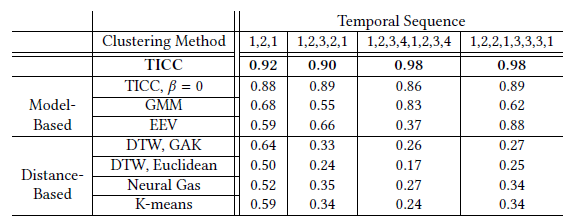TICC算法（蓝方块）聚类所需的样本数也较少。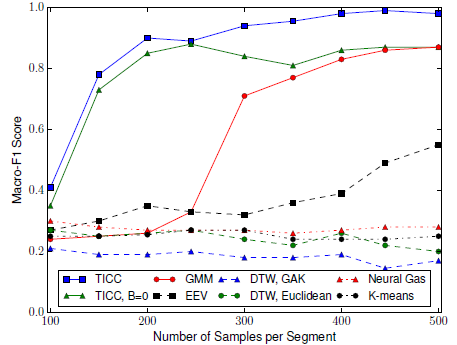可扩展性
使用n=50n=50的高维样本，聚类数量K=5K=5，窗口宽度w=3w=3。下图示出，当信号长度增加时，聚类时间的增长基本为线性。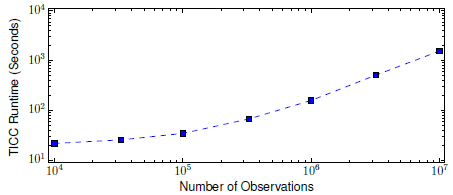真实案例
给出了汽车行驶案例，大家体会一下。
传感器n=7n=7
刹车状态
前向加速度
侧向加速度
方向盘角度
速度
发动机转速
油门状态
每一组观测时长1小时，每隔0.1秒采样一次。共有36000组观测。
使用的窗口w=10w=10时长1秒。
使用BIC方法3发现的最佳聚类数量为K=5K=5。人工观察，它们对应于如下状态：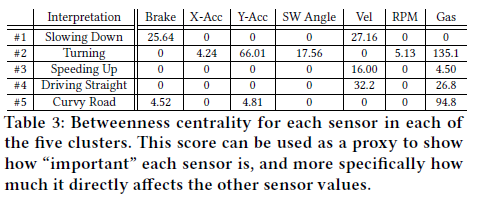此处待推导。思路：证明XiTΘXiX_i^T\Theta X_i是S⋅ΘS\cdot \Theta的特征值。其总和为该矩阵的迹。 ↩︎

S. Boyd, N. Parikh, E. Chu, B. Peleato, and J. Eckstein. Distributed optimization and statistical learning via the alternating direction method of multipliers. Foundations and Trends in Machine Learning, 2011. ↩︎

T. Hastie, R. Tibshirani, and J. Friedman. The Elements of Statistical Learning. Springer, 2009. ↩︎


展开全文算法 clustering
• python 平滑时间序列In time series analysis, the presence of dirty and messy data can alter our reasonings and conclusions. This is true, especially in this domain, because the temporal dependency ...java 人工智能 机器学习 leetcode
• 主要针对控制图时间序列数据集的聚类任务，使用了基于划分的（K-Means）、基于层次的（AGNES）、基于密度的（DBSCAN）以及基于图的（spectral clustering）聚类方法，最后可视化结果，用Jupyter Notebook编写...
• 目前有一用电数据集（txt格式），想用kmeans实现对该时间序列的聚类。 但kmeans是针对单个数据点的聚类，而不是曲线...**急求大佬给改进意见，或者直接扔给我matlab/python的能实现时间序列聚类的Kmeans代码，跪谢。**数据挖掘 机器学习
• 目的：对波形数据或时间序列数据进行聚类。 tslearn是基于python的机器学习库之一。 tslearn： : 用日语。 使用KShape算法对样本数据执行波形聚类。 必须为算法指定簇数作为参数。这次，我预先检查了数据，并...
• 时间聚类分析，TCA算法的思想就是确定在一个序列图像上所有点是在什么时间出现变化（响应）极大值（极小值），而忽略其他细节。 1.最近邻分析 利用各个点与其最近邻点的距离，判断该点是呈随机的、规则的还是聚集...
• 它使用通用API结合了Python的ScikitLearn（通过其互补的软件包）和Julia ML的ML库，并允许无缝集成和集成异构ML库以创建复杂的模型，以进行可靠的时间序列预测。 该包的设计/框架受Samuel Jenkins的和包的影响很大...
• 该库允许基于Reservoir Computing（由Echo State Networks在机器学习中普及的方法系列）快速实现不同的体系结构，以对单变量/多元时间序列进行分类或聚类。 通过为每个模块选择不同的配置，可以使用几个选项来自...
• n)”加载时间序列的帧“n”。 计算光流 使用 计算光流。 Lucas-Kanade 方法假设局部空间和时间图像梯度可以建模为两个相邻帧之间强度的线性转换。 此处的光流计算使用高斯导数 (DoG) 内核来计算空间和时间图像梯度，...
• 该工具箱可根据时间序列的频谱特性对其进行聚类，对比和分类。 它由具有执行每个步骤的代码的子模块组成。 2.哪里 根目录有两个子目录：src和docs。 src包含模块和子模块的所有源代码。 docs包含所有文档。 每个模块...
• 最近在做一个时空序列聚类的小项目，度量聚类优劣的指标使用到了DBI指数，所以开始学习了一下...首先假设我们有m个时间序列，这些时间序列聚类为n个簇。m个时间序列设为输入矩阵X，n个簇类设为N作为参数传入算法。使...数据挖掘
• em算法matlab代码Python代码用于功能数据的聚类和细分，并结合了回归与隐藏逻辑流程（MixRHLP）模型： << Github上还提供了R和Matlab版本>> 由编写的python代码 Faicel Chamrouckhi和Marius Bartcus 运行我们...
• 用于能源负载时间序列Python工具包 enlopy是一个开放源代码python库，包含用于生成，处理，分析和绘制时间序列的方法。 尽管它可以用于任何类型的数据，但它都非常注重与能源有关的数据，例如电/热需求或发电量...
• 树状图时间序列 Scipy的聚类聚类树状图要求进行广泛的自定义，以使其信息更丰富。 此程序包通过两个自定义包装scipy的树状图： 侧面的时间序列图 距离标签和聚类分裂点 安装 下载dendrogram_ts.py并放入您的python ...
• 人工智能的基本概念 Python的人工智能入门 Python机器学习 ... Python分析时间序列数据 Python语音识别 Python启发式搜索 Python游戏 基于Python神经网络 Python强化学习 Python遗传算法
• DTW广泛用于例如计量经济学，化学计量学和常规时间序列挖掘中的分类和聚类任务。 该软件包提供了最新的动态时间规整类型（DTW）算法的最完整，可自由使用（GPL）的实现。 它是C上的忠实Python等效项。 支持任意...
• 该软件提供了一个代码框架，可从时间序列（或时间序列数据集）中提取成千上万的时间序列分析功能，以及用于对数据进行规范化和聚类，生成数据的低维表示的工具，识别不同时间序列类别之间的区分特征，使用大量时间...
• AI与Python教程导航 人工智能的基本概念 Python的人工智能入门 Python机器学习 Python数据准备 Python监督学习：分类 Python监督学习：回归 ... Python分析时间序列数据 Python语音识别 ...
• 人工智能的基本概念 Python的人工智能入门 Python机器学习 ... Python分析时间序列数据 Python语音识别 Python启发式搜索 Python游戏 基于Python神经网络 Python强化学习 Python遗传算法
• 一个兼容的Java工具箱，用于时间序列分类，聚类和转换。 有关python sklearn兼容版本的信息，请参见 在网站上找到有关UCR单变量和UEA多元时间序列分类档案的广泛工作和数据集托管的更多信息。 正在为我们的研究积极...
• 学会的结构通常可以将函数分解为可解释的组件，并可以对时间序列数据集进行长期外推。 在各种预测任务上，我们的结构搜索方法优于许多广泛使用的内核和内核组合方法。 如有任何问题，请随时给我们发送电子邮件： ...# python时间序列聚类python 订阅